﻿ Mechanics Map - Cross Product

# Cross Product

The cross product is a mathematical operation that can be performed on any two, three dimensional vectors. The result of the cross product operation will be a third vector that is perpendicular to both of the original vectors and has a magnitude of the first vector times the magnitude of the second vector times the sine of the angle between the vectors.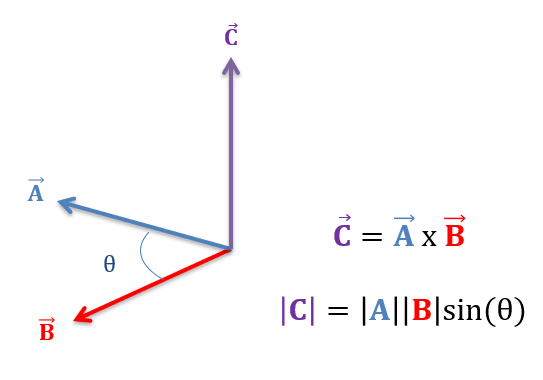The cross product of two vectors will be a vector that is perpendicular to both original vectors with a magnitude of A times B times the sine of the angle between A and B.

When finding a cross product you may notice that there are actually two directions that are perpendicular to both of your original vectors. These two directions will be in exact opposite directions. To find which of these two directions the cross product uses, we will use the right hand rule.

To use the right hand rule, hold out you right hand, point your index finger in the direction of the first vector, turn your middle finger in towards the direction of the second vector, and hold your thumb up. Your thumb should now point in the direction of the cross product vector.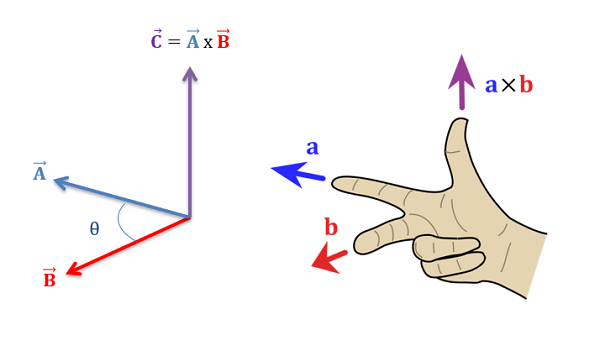We can use the right hand rule to determine the direction of the cross product. Image adapted from work by Acdx licensed under a CC-BY-SA 3.0 licensee.

One additional thing you can note with the right hand rule is that switching the order of the two input vectors (switching A and B) would result in the cross product pointing in exactly the opposite direction. This is because the cross product operation is not communicative, meaning that order does matter. Specifically, switching the order of the inputs gives you a result that is exactly the opposite of what your original calculation.

## Calculating the Cross Product

To find the cross product by hand, the easiest method is as follows.

1. Write out the letters, x y z x y in a row as shown in the diagram below.
2. Write out the x, y, and z components of the first vector underneath the corresponding letters of above. Repeat this for the second vector, writing out the second vector in a row under the first vector.
3. Draw in diagonals as shown in the diagram. The diagonals that travel to the right as they move down represent positive quantities while the diagonals that travel to the left as they move down represent negative quantities.
4. Using the letters the diagonals travel through in the top row as a guide for which component of the result each quantity is part of take the sum of the positive and negative diagonal products for each of the three components in the result. This should give you the final formula shown in the diagram.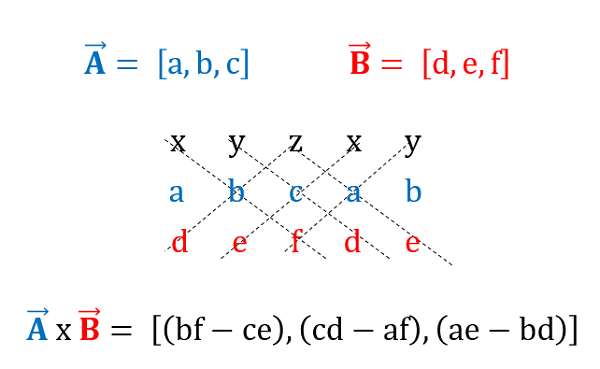We can use the visual aid above to help remember the formula for the cross product.

In addition to calculating the cross product by hand we can also use computer tools such as the "cross" command in MATLAB or web based tools such as the Wolfram Vector Operation calculator linked to in the sidebar of this page. Access to these tools allows you to very easily and quickly calculate the cross product and is a major advantage to using vectors operations to analyze problems.

## Worked Problems:

### Question 1:

Calculate the cross product of force vectors A and B in the diagram below by hand.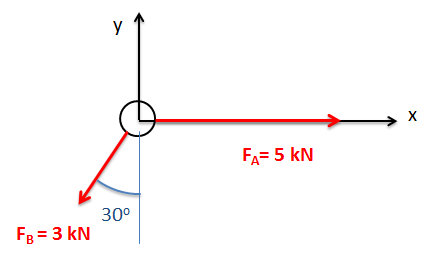### Question 2:

Calculate the cross product of vectors A and B in the diagram below by hand.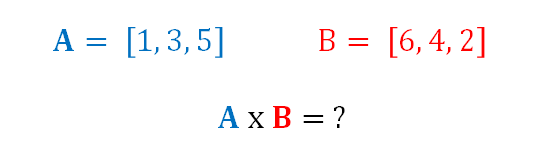### Question 3:

Calculate the cross product of vectors A and B in the diagram below using MATLAB.### Question 4:

Calculate the cross product of vectors A and B in the diagram below using the Wolfram Vector Operation Calculator.